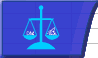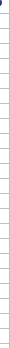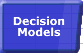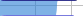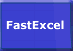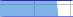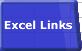# 'Calculate' always in the Status BarSmart Recalculation Evaluation Circumstances Calculation Process Dependency Trees Controlling Calculation "CALCULATE" in Status Bar Calculation Methods Calculating from VBA Volatile Functions & Actions User-Defined Functions Repetitive Calculation Version Timing Comparison### Status Bar Shows CALCULATE

There are six known conditions in which the status bar will show CALCULATE:

• The Calculation Option has been set to Manual and the workbook contains uncalculated formulae. Try setting calculation to Automatic (Tools-->Options-->Calculate). Note that Excel sets the calculation mode from the first workbook opened in a session: when you open two workbooks, one saved in manual mode and one saved in automatic mode, they will both have the calculation mode of the first workbook opened.
• The Iteration Option is turned on and the workbook contains circular references. Check that turning off Iteration (Tools-->Options-->Calculation) and pressing F9 shows "Circular Reference" in the statusbar.
• You are using Excel 2000 without the SR1 update and have a user-defined function that attempts to define a name and depends on a volatile function: see MSKB Q248179
• You have hit one of Excels limits for tracking dependencies.
• You are using Excel 2007 or later and have set Workbook.ForceFullCalculation to True
• You are using Excel 2013 or later and have multiple windows open. See my blog post excel 2013 sdi bug calculate in status bar strikes again

## Dependency Tree Limits

There are two limits to the number of dependencies that Excel versions prior to Excel 2007 can track before it must do full calculations instead of recalculations.

• The number of different areas in a sheet that may have dependencies is limited to 65,536.
• The number of cells that may depend on a single area is limited to 8K.

After the workbook has passed these limits, Excel no longer attempts to recalculate only changed cells. Instead, it recalculates all cells at each calculation. These limits saves the substantial amount of memory required for the dependency trees. When this happens the status bar permanently shows CALCULATE for all open workbooks even after closing the main workbook, until you close and reopen Excel.

If you find that recalculation is taking longer than full calculation, or the time taken to update the dependency tree at each change is too large, you can force each calculation to be a full one using Workbook.ForceFullCalculation from VBA.

I do not know of a way of counting the number of dependencies, so it is not easy to work out how to get below the limit, but:

• Large numbers of dependencies are usually caused by many formulae referencing large ranges using functions like OFFSET, INDEX, and the Lookup. You can reduce the number of dependencies by reducing the use of these functions, and also by reducing the size of the ranges they refer to. If you reduce the number of dependencies below the limits you have to save the workbook, close and restart Excel before Excel recognises that the number of dependencies has fallen below the limits.
• Excel 2002 allows you to do a full recalculation with dependency tree rebuild, but this does not alter the limits.
• When you have exceeded the dependency limitspressing F9 will cause a recalculation even when in Automatic mode.

Because there are other conditions that can cause "Calculate" to appear in the status bar, test for the other conditions::

• Turn off Iteration, press F9 and look for "Circular Reference" in the statusbar.
• Press Ctrl/Alt/F9 to do a full calculation. If you have hit the limit the status bar will show the calculation % climbing to and reaching 100%, and then being replaced by "Calculate"

For Microsoft's official view on the dependency limit see MSKB Article Q243495 "Calculate Message Remains in Status Bar If 65,536 Formula References"© 2001-2020 Decision Models Legal Disclaimer Privacy Statement